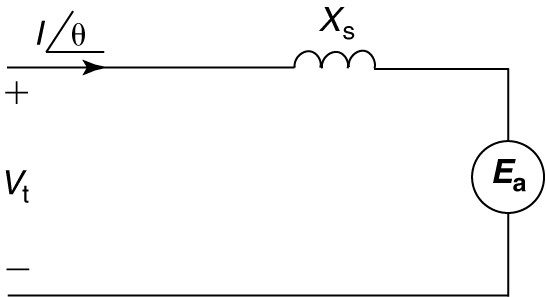Home / Synchronous Machines / Synchronous Motor: Equivalent Circuit & Phasor Diagram

# Synchronous Motor: Equivalent Circuit & Phasor Diagram

Want create site? Find Free WordPress Themes and plugins.

The equivalent circuit for one phase of a synchronous motor is shown in Figure 1. It is similar to the synchronous generator equivalent circuit, but with two differences.

First, it has been flipped to show the power system as the input to the motor, and, second, the current is referenced as positive into the motor.

The power system normally constitutes an infinite bus because the motor is much smaller than the rest of the power system. When the rotor field rotates at synchronous speed, a counter EMF (C-EMF), Ea, is induced into the armature windings. Writing a voltage loop equation yields

$\begin{matrix} {{V}_{t}}={{E}_{a}}+jI{{X}_{s}} & {} & \left( 1 \right) \\\end{matrix}$

Which can be rearranged as

$\begin{matrix} {{E}_{a}}={{V}_{t}}-jI{{X}_{s}} & {} & \left( 2 \right) \\\end{matrix}$FIGURE 1:  Per-phase equivalent circuit for a synchronous motor.

## Synchronous Motor Phasor Diagram

Equation 2 is the preferred form since the terminal voltage is normally chosen as the reference phasor for purposes of drawing a phasor diagram. With a known terminal voltage, we can construct phasor diagrams for the synchronous motor, as shown in Figure 2. Figure 2(a) shows a phasor diagram for a lagging current.FIGURE 2:  Phasor diagrams for a synchronous motor.

1. Lagging current.
2. Leading current.

Since the motor is a load, a lagging current means the motor is accepting lagging current and is thus acting like an inductive load. The voltage drop across the synchronous reactance is -jIXs. The -j operator constitutes a rotation of 90° in the clockwise direction, so the synchronous reactance voltage drop is perpendicular to the current in the direction shown.

The resultant C-EMF is found to be lagging the terminal voltage by the power angle delta (δ). From the phasor diagram in Figure 2(a) we can observe that

$\begin{matrix} {{E}_{a}}\cos \delta <{{V}_{t}} & {} & \left( 3 \right) \\\end{matrix}$

Which means the motor is operating in an under-excited condition, drawing reactive power from the infinite bus.

Figure 2(b) shows a phasor diagram for a motor operating with a leading current. Since the motor is accepting leading current, it is acting like a capacitive load. In this case, the generated voltage still lags the terminal voltage by the power angle, but the magnitude of the generated voltage is now greater than the terminal voltage, or, more specifically,

$\begin{matrix} {{E}_{a}}\cos \delta >{{V}_{t}} & {} & \left( 4 \right) \\\end{matrix}$

In this case, the motor is overexcited and provides reactive power to the infinite bus.

Synchronous motors are typically rated to deliver a certain horsepower while operating at a particular value of power factor.

The most common power factor ratings for synchronous motors are unity and 0.8. A motor rated for 0.8 power factor would obviously be larger and more expensive than one rated for unity power factor because both the armature conductors and the field conductors would have to carry more current at the lower power factor.

If operated at less than unity power factor, the synchronous motor would almost certainly operate in the leading direction.

Did you find apk for android? You can find new Free Android Games and apps.

### About Ahmad FaizanMr. Ahmed Faizan Sheikh, M.Sc. (USA), Research Fellow (USA), a member of IEEE & CIGRE, is a Fulbright Alumnus and earned his Master’s Degree in Electrical and Power Engineering from Kansas State University, USA.